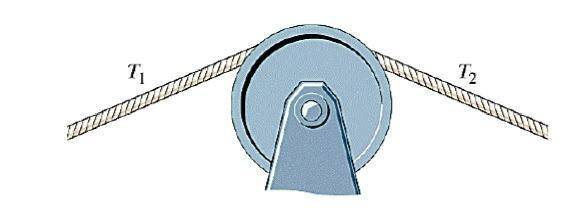# A string over a pulley, same tension?

Hi, Im new here!

1. There is no actually data given, Im just supposed to explain why a string that crosses a pulley have the same tension both sides. The pulleys moment of inertia is zero and friction is negligible.
There is a little hint in this problem that says; study equation 9-15, which is:"Στ=lα"The only thing I know here is that a pulley doesnt change the tension just the direction.

Kate

Hi! Try to imagine the situation when you attach one body (mass m) to both ends of a string hung up by a pulley. Describe this system from the point of view of dynamics (Newton's II. Law). Important thing to note: you can also write Newton's Laws for different cross-sections of the string. This will probably lead you to the answer. I hope I could help a little :)

Hi! Try to imagine the situation when you attach one body (mass m) to both ends of a string hung up by a pulley. Describe this system from the point of view of dynamics (Newton's II. Law). Important thing to note: you can also write Newton's Laws for different cross-sections of the string. This will probably lead you to the answer. I hope I could help a little :)

I still dont understand how I should explain why the tension must be the same?

Maybe my help was a bit complicated. Let's try a simpler problem. No pulleys yet. You attach one end of a rope to the ceiling and the other end to a body of let's say 5 kg. The force that is required to hold this (balance the gravitational force/weight) body will be the tension in the rope, because the rope is exerting this force. You can write Newton's II. Law for the body. Two forces: weight and tension. Their vectorial sum is the net force, and because the body is not moving that must be zero. To be able to prove that the tension is equal everywhere in the rope you have the consider a part of the rope as a different 'body" for which you can also write Newton's II. Law. Note: two forces again, net force zero. If you got this, try to apply this method for a system with a pulley.

Maybe my help was a bit complicated. Let's try a simpler problem. No pulleys yet. You attach one end of a rope to the ceiling and the other end to a body of let's say 5 kg. The force that is required to hold this (balance the gravitational force/weight) body will be the tension in the rope, because the rope is exerting this force. You can write Newton's II. Law for the body. Two forces: weight and tension. Their vectorial sum is the net force, and because the body is not moving that must be zero. To be able to prove that the tension is equal everywhere in the rope you have the consider a part of the rope as a different 'body" for which you can also write Newton's II. Law. Note: two forces again, net force zero. If you got this, try to apply this method for a system with a pulley.

So the tension in the string must be the same because? Ive done the example you described it but I cant figure out a good way to explain it.

What did you get for the forces on a part of the rope?

What did you get for the forces on a part of the rope?
what do you mean by: "for the forces"?

what do you mean by: "for the forces"?
In order to prove that the tension in the rope is equal everywhere, you have to describe the forces acting on a cross section of the rope.
I think the best way I could help would be if you told be how did you attack this problem. How would you start?

haruspex
Homework Helper
Gold Member
2020 Award
1. There is no actually data given, Im just supposed to explain why a string that crosses a pulley have the same tension both sides. The pulleys moment of inertia is zero and friction is negligible.
There is a little hint in this problem that says; study equation 9-15, which is:"Στ=lα"

The only thing I know here is that a pulley doesnt change the tension just the direction.
Welcome to PF.
First, let me clarify one thing. When a problem regarding pulleys says ignore friction it generally means friction at the axle, not friction between pulley and rope. If there's no friction between pulley and rope you don't need it to be a pulley at all.
Answering this question in detail is a bit tricky. In principle, the tension could vary along the part of the rope in contact with the pulley. If we consider that made up of many short sections, each section could create a net torque, namely, the difference in the tensions at the ends of the section multiplied by the radius. The torque from the tension at one end of such a section cancels the torque from the tension at the adjoining end of the next section. So when these are all added up you are left with the two torques at the points where the straight rope sections meet the pulley.
Suppose thesewo tensions are different. What can you say about the net torque? What does the equation in your hint then tell you?